# Question about the energy stored in an RLC parallel resonant network

• cianfa72
In summary, the parallel resonant circuit has zero phase angle between the sinusoidal voltage source (emf) and the current through the inductor, while the series resonant circuit has an 'phase angle' between the sinusoidal voltage source (emf) and the current through the inductor.f

#### cianfa72

TL;DR Summary
The total energy stored in a RLC resonant network feeds from a sinusoidal source should stays at steady-state constant in time
Hi,

I've a doubt about how to the energy is stored in a 'real' RLC parallel resonant network feeds from a sinusoidal source. Take a 'real' RLC parallel network having a resistor ##R_s## in series with the inductor ##L_s## (modeling its loss) with the capacitor C in parallel and consider it in steady-state at resonance.

Analyzing the network doing a series-parallel transformation of the couple ##R_s## and ##L_s## into ##R_p## and ##L_p## we can see that the overall energy stored in the RLC network stays constant in time. To prove it just use the following formulas for the Energy of the inductor and capacitor

## E_L(t) = \frac 1 2 L i_L^2(t)## and ## E_C(t) = \frac 1 2 C v_C^2(t)##

then doing the job at ##\omega = \omega_r = \sqrt { \frac 1 {L_sC} - \frac {R^2_s} {L^2_s} }## we can check actually ##E(t) = E_L(t) + E_C(t) = const##

I tried to do the calculation without involving the series-parallel transformation (basically working on the initial RLC network), however this time it seems the total energy stored in the inductor + capacitor is no more constant in time.

The 'problem' here is that we have not anymore a sum of the type ##sen^2(\omega_rt) + cos^2(\omega_rt) = 1## because this time there is an 'phase angle' between the sinusoidal voltage source (emf) and the current through the inductor ##L_s##.

If you want I can add the detail of my job...thank you

Last edited:
Summary:: The total energy stored in a RLC resonant network feeds from a sinusoidal source should stays at steady-state constant in time

Hi,

I've a doubt about how to the energy is stored in a 'real' RLC parallel resonant network feeds from a sinusoidal source. Take a 'real' RLC parallel network having a resistor ##R_s## in series with the inductor ##L_s## (modeling its loss) with the capacitor C in parallel and consider it in steady-state at resonance.

Analyzing the network doing a series-parallel transformation of the couple ##R_s## and ##L_s## into ##R_p## and ##L_p## we can see that the overall energy stored in the RLC network stays constant in time. To prove it just use the following formulas for the Energy of the inductor and capacitor

## E_L(t) = \frac 1 2 L i_L^2(t)## and ## E_C(t) = \frac 1 2 C v_C^2(t)##

then doing the job at ##\omega = \omega_r = \sqrt { \frac 1 {L_sC} - \frac {R^2_s} {L^2_s} }## we can check actually ##E(t) = E_L(t) + E_C(t) = const##

I tried to do the calculation without involving the series-parallel transformation (basically working on the initial RLC network), however this time it seems the total energy stored in the inductor + capacitor is no more constant in time.

The 'problem' here is that we have not anymore a sum of the type ##sen^2(\omega_rt) + cos^2(\omega_rt) = 1## because this time there is an 'phase angle' between the sinusoidal voltage source (emf) and the current through the inductor ##L_s##.

If you want I can add the detail of my job...thank you
I think you have come across the issue which we discussed on this forum a few weeks back, that for the parallel resonant circuit (using R and L in series), max impedance and zero phase angle do not coincide. The resonant frequency is that where phase angle between applied voltage and current is zero. In this case, at switch-on the stored energy will build up asymptotically over several cycles and then remain constant, and the generator will then only supply the resistor. At switch-off, the stored energy will be transferred to the resistor over several cycles.

Take a 'real' RLC parallel network having a resistor ##R_s## in series with the inductor ##L_s## (modeling its loss) with the capacitor C in parallel and consider it in steady-state at resonance.
What you have described is a "real" series resonant circuit. A short version of the series parallel distinction is this: Imagine your an electron at one side of the resistor and you want to travel to the other side. If you have to go through both the L and C, then it's a series circuit. If you could choose L or C and only go through one, then it is a parallel circuit.

Analyzing the network doing a series-parallel transformation of the couple Rs and Ls into Rp and Lp
Sorry, I didn't follow this. How do you transform a series Rs-Ls (which has Z(jω) → Rs as ω → 0 and Z(jω) → ∞ as ω → ∞) into a parallel Rp-Lp (which has Z(jω) → 0 as ω → 0 and Z(jω) → Rp as ω → ∞)?

I'll leave the energy stuff for you to work out. But, it may help to just look at the impedance of the series circuit vs. the parallel circuit as seen by the source:

First, let s≡jω, so, for example, the impedance of the inductor is Z(s)=sL=jωL

For the series RLC: ## Z_s(s) = R + sL + \frac{1}{sC} = \frac{1 + sCR + {s^2}LC}{sC} ##

For the parallel RLC: ##
Z_p(s) = \frac{1}{\frac{1}{R} + \frac{1}{sL} + sC} = \frac{sL}{1 + s\frac{L}{R} + {s^2}LC} ##

It is convenient to express these in a canonical form like this:
## P(s) \equiv 1 + (\frac{1}{Q})( \frac{s}{\omega_o}) + (\frac{s}{\omega_o})^2## with ## \omega_o \equiv \frac{1}{\sqrt{LC}} ## and ## Z_o \equiv \sqrt{\frac{L}{C}} ##

Then, the series impedance is ##Z_s = \frac{P(s)}{sC} ## with ## Q = \frac{Z_o}{R} ##

And, the parallel impedance is ##Z_p = \frac{sL}{P(s)} ## with ## Q = \frac{R}{Z_o} ##

Then at resonance ##(\frac{s}{\omega_o}) =j## and ## P(s) = (\frac{j}{Q}) ## in both cases there is no phase shift at resonance.

The symmetry here makes these easier to remember and understand the more general issues. I find it easiest to keep the two forms straight by thinking about what the impedance is, in each case, at ω → 0 and ω → ∞.

edit: fixed typos

Last edited:
What you have described is a "real" series resonant circuit. A short version of the series parallel distinction is this: Imagine your an electron at one side of the resistor and you want to travel to the other side. If you have to go through both the L and C, then it's a series circuit. If you could choose L or C and only go through one, then it is a parallel circuit.
It is a tricky area but I don't think that is quite right because his generator is not across R. It is at the generator that the properties of the tuned circuit are being studied.

I think you have come across the issue which we discussed on this forum a few weeks back, that for the parallel resonant circuit (using R and L in series), max impedance and zero phase angle do not coincide. The resonant frequency is that where phase angle between applied voltage and current is zero.
That's right, however I do not understand how it might be related to the Energy stored in the system (inductor + capacitor) in AC steady-state at RLC network resonant angular frequency ##\omega_r = \sqrt { \frac 1 {L_sC} - \frac {R^2_s} {L^2_s} }##

In this case, at switch-on the stored energy will build up asymptotically over several cycles and then remain constant, and the generator will then only supply the resistor. At switch-off, the stored energy will be transferred to the resistor over several cycles.
As you said at AC steady-state the energy stored (into inductor + capacitor) should remain constant in time; that's my problem doing the calculation for the given circuit. Maybe I did some mistake...Sorry, I didn't follow this. How do you transform a series Rs-Ls (which has Z(jω) → Rs as ω → 0 and Z(jω) → ∞ as ω → ∞) into a parallel Rp-Lp (which has Z(jω) → 0 as ω → 0 and Z(jω) → Rp as ω → ∞)?
The point is that you can transform the R-L series in an R-L parallel at a given angular frequency ##\omega##. Thus we can actually do the transformation having ##R_p(\omega)## and ##L_p(\omega)## as functions of ##\omega## (see the picture below)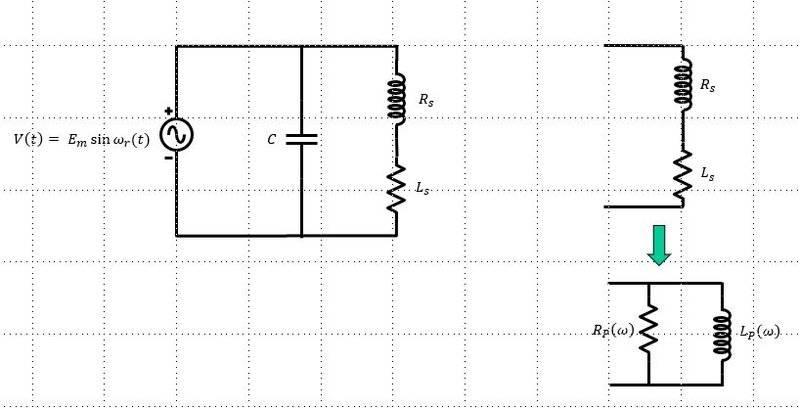The issue I'm facing to is that in the second case (transformed one) the total energy stored in AC steady state in the RLC network at resonant angular frequency ##\omega_r## is constant in time (as supposed to be), however doing the calculation for the initial network (no transformation) it seems it is not constant in time anymore...

Edit: The calculation of the total stored energy in AC steady-state at frequency ##\omega_r## for the initial network (no ##R_s## - ##L_s## transformation) gives:

##E(t) = \frac 1 2 L_s i_L^2 (t) + \frac 1 2 C v_C^2(t) = \frac 1 2 C E^2_m [sin^2 (\omega_rt + \phi) + sin^2 (\omega_rt)]##

with phase angle ##tan(\phi) = - \frac {\omega_rL_s} {R_s}##

I believe the above quantity ##E(t)## actually does not remain constant in time, though...

#### Attachments

Last edited:
Thanks for posting the schematic, now I know what we are actually talking about and can (hopefully) give some useful comments.

I've a doubt about how to the energy is stored in a 'real' RLC parallel resonant network feeds from a sinusoidal source. Take a 'real' RLC parallel network having a resistor Rs in series with the inductor Ls (modeling its loss) with the capacitor C in parallel and consider it in steady-state at resonance.

This is not a resonant system. It would be if the voltage source was removed, or changed into a current source. When you add the voltage source you are changing the network by adding a zero impedance branch across the capacitor. So, the capacitor voltage isn't dependent on any of the other circuit parameters; it doesn't matter what is happening to the L-R branch if you only care about the capacitor voltage/current. You could just as well split this into two separate networks for analysis as shown in the photo below.

For more advanced readers, it isn't a second order system because it only has one state variable, the inductor current (or equivalent). The capacitor voltage isn't a state variable since it is determined by the driving function. In general, loops with only capacitors and voltage sources, or branches with only inductors and current sources, are degenerate.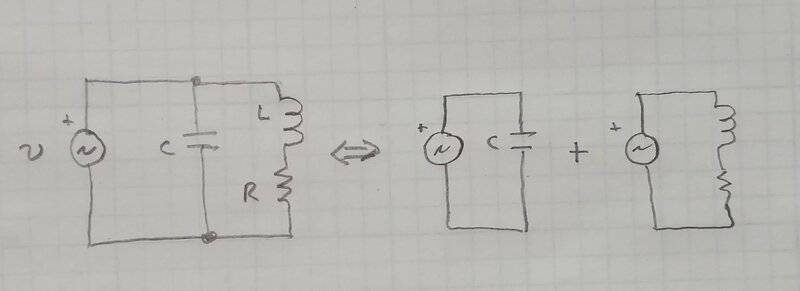Analyzing the network doing a series-parallel transformation of the couple Rs and Ls into Rp and Lp we can see that the overall energy stored in the RLC network stays constant in time.

Um... no. That's not a circuit analysis thing. Whoever taught you to do that wasn't doing you any favors. I think what you should have said is:
"Analyzing the Creating a new, different, network doing a series-parallel transformation of the couple Rs and Ls into Rp and Lp we can see that the overall energy stored in the new RLC network stays constant in time."

For example, let's look at the inductor voltage in the two networks. Should it depend on the resistor voltage/current? In the first it does, in the second it is determined solely by the voltage source. Or, you could break down the new network into three separate problems similar to the phot above.

Yes, the new network is easier to solve. This is quite irrelevant vis-a-vis the original problem.

then doing the job at ω=ωr=1LsC−Rs2Ls2 we

We would normally define the resonant frequency independent of R, as I did in post #3. Except for this problem, which doesn't have one (see above).

I tried to do the calculation without involving the series-parallel transformation (basically working on the initial RLC network), however this time it seems the total energy stored in the inductor + capacitor is no more constant in time.

The 'problem' here is that we have not anymore a sum of the type sen2(ωrt)+cos2(ωrt)=1 because this time there is an 'phase angle' between the sinusoidal voltage source (emf) and the current through the inductor Ls.

Yes, good! You are correct (although we haven't seen the details of your work). The stored energy isn't constant when you add in the resistance, because of the phase shift added, as you correctly said. Consider the case where the resistance is increased to a large value compared to the L & C, then there will be little inductor current to "smooth out" the energy fluctuation in the capacitor. It's not a 'problem' it's how these things really are.

Did I make some mistake in the calculation or not ?
We haven't seen your calculations, but probably not. Your mistake is a combination of incorrect assumptions about the answer you are seeking, and a fundamentally incorrect network transformation.

Finally, you can do network transformations to create dual networks. But you have to follow specific rules, must importantly, EVERYTHING gets transformed; loops become nodes, nodes become loops; inductors become capacitors; voltage sources become current sources, etc. Personally I think it's nearly useless as an analysis tool, the problem and solution remains exactly the same.

This is the correct dual network transformation: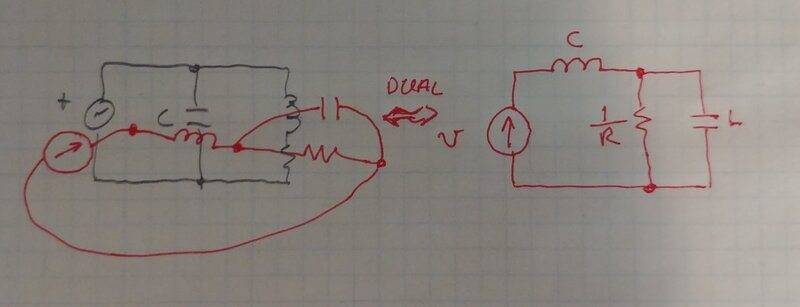Last edited:
In LCR resonant circuits, the total energy doesn't fluctuate at ωo (that would create serious energy conservation questions), although it does decay at a rate determined by the losses. This is different from your circuit because the voltage drop across the resistor effects both the L & C.

I think the problem you were meant to solve was one of these: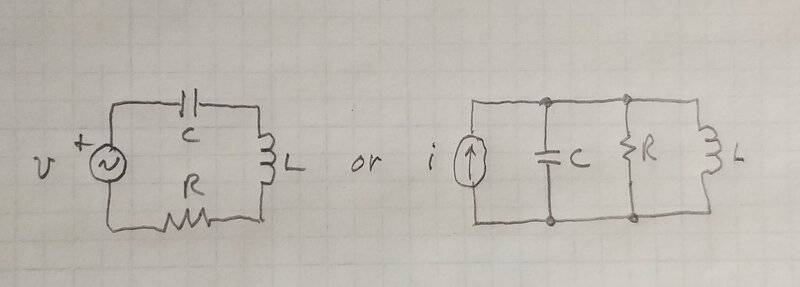Last edited:
This is not a resonant system. It would be if the voltage source was removed, or changed into a current source. When you add the voltage source you are changing the network by adding a zero impedance branch across the capacitor. So, the capacitor voltage isn't dependent on any of the other circuit parameters; it doesn't matter what is happening to the L-R branch if you only care about the capacitor voltage/current. You could just as well split this into two separate networks for analysis as shown in the photo below.

For more advanced readers, it isn't a second order system because it only has one state variable, the inductor current (or equivalent). The capacitor voltage isn't a state variable since it is determined by the driving function. In general, loops with only capacitors and voltage sources, or branches with only inductors and current sources, are degenerate.

If we calculate the impedance of the circuit in post #5, and let the voltage source be 10 volts AC swept in frequency from 2000 Hz to 10000 Hz, we can calculate the current drawn from the source, and plot that current. Here is the plot with R=100, L=.1, and C= .01 uF: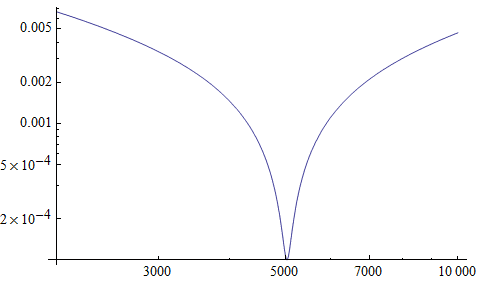It sure looks like what one would expect to get if the system were second order. How do we get a response like this if the system isn't second order?

If we calculate the impedance of the circuit in post #5, and let the voltage source be 10 volts AC swept in frequency from 2000 Hz to 10000 Hz, we can calculate the current drawn from the source, and plot that current. Here is the plot with R=100, L=.1, and C= .01 uF:

View attachment 283056

It sure looks like what one would expect to get if the system were second order. How do we get a response like this if the system isn't second order?
Yes the impedance is second order, similar to post #3, ## Z(s) = R \frac{(1+s\frac{L}{R})}{(1+ sCR + s^2LC)} ##.

But I don't think the system is second order because of the zero impedance voltage source that is shunting the capacitor. Again, the capacitor voltage depends only on the instantaneous value of the voltage source, it does not represent any of the past history of the circuit, it's value does not depend on any of the circuit's dynamic response. The only "memory" in this system is the inductor current, which is the integral (i.e. history) of the inductor voltage. Current that otherwise might flow through the capacitor and be integrated to appear as the voltage is shunted through the source in the exact amount to control the capacitor voltage to match the present conditions. Also, notice that the system can only have one initial condition, the inductor current. If you set an initial condition on the capacitor, it will be instantly changed (with ∞ current) at t=0.

In the system DEs where you would otherwise see ## \frac{1}{C} \int {i_l}dt = L \frac{di_l}{dt} + Ri_l ##, we instead get ## V(t) = L \frac{di_l}{dt} + Ri_l ## and ## i_c = C \frac{dV(t)}{dt} ##, two uncoupled systems, one first order and one zero order. Although I probably wouldn't argue too much if you wanted to call the capacitor loop a first order system.

OTOH, I think I was probably wrong to say the system isn't resonant. For high Q the inductor and capacitor currents do cancel at ##\omega_o##.

edit: Oops! Sign error, should be: ## \frac{1}{C} \int {i_l}dt = -L \frac{di_l}{dt} - Ri_l ##

Last edited:
Yes the impedance is second order, similar to post #3, .

But I don't think the system is second order because of the zero impedance voltage source that is shunting the capacitor. Again, the capacitor voltage depends only on the instantaneous value of the voltage source, it does not represent any of the past history of the circuit, it's value does not depend on any of the circuit's dynamic response. The only "memory" in this system is the inductor current, which is the integral (i.e. history) of the inductor voltage.
I believe the point is that the transfer function ## F(s) = \frac {I(s)} {E(s)} ## -- where ##E(s)## is the Laplace transform of the applied voltage source and ##I(s)## the current throught it -- actually has a couple of complex conjugate zeros and not poles !

Even if the above transfer function evaluated at ##s=j\omega_r## has zero phase, that does not actually means the network has got a resonance at angular frequency ##\omega_r##.

In the system DEs where you would otherwise see ## \frac{1}{C} \int {i_l}dt = L \frac{di_l}{dt} + Ri_l ##, we instead get ## V(t) = L \frac{di_l}{dt} + Ri_l ## and ## i_c = C \frac{dV(t)}{dt} ##, two uncoupled systems, one first order and one zero order.
Yes, I see: we have two uncoupled Differential Equations (DEs) or in other words -- as you said -- the network actually splits in two separate uncoupled networks.

Last edited:
We haven't seen your calculations, but probably not.
This is my job:

##\omega_r = \sqrt { \frac 1 {L_sC} - \frac {R^2_s} {L^2_s} }##

##v_g(t) = E_msin(\omega_rt)##

##i_c(t) = E_m\omega_rCcos(\omega_rt)##

##i_L(t) = \frac {E_mR_s} {R^2_s + \omega^2_rL^2_s} sin(\omega_rt) - \frac {E_m\omega_rL_s} {R^2_s + \omega^2_rL^2_s}cos(\omega_rt) = \frac {E_mR_sC} {L_s}sin(\omega_rt) - E_m\omega_rCcos(\omega_rt)##

##i_g(t) = i_c + i_L = \frac {E_mR_sC} {L_s}sin(\omega_rt)##

##P(t) = v_g(t)i_g(t) = \frac {E^2_mR_sC} {L_s}sin^2(\omega_rt)##

then for the instantaneous power dissipated on the resistor ##R_s## I get:

##W(t) = R_si^2_L(t) = \frac {E^2_m} {R^2_s + \omega^2_rL^2_s}sin^2(\omega_rt + \phi) = \frac {E^2_mR_sC} {L_s}sin^2(\omega_rt + \phi)##

##tan(\phi) = - \frac {\omega_rL_s} {R_s}##

We can see that the energy ##P(t)dt## from the voltage generator evaluated at time t is not the same as the energy ##W(t)dt## dissipated on the resistor ##R_s## at the same time (even if maximum values of both ##P(t)## and ##W(t)## are the same). Maybe this difference at time t basically accounts for the "excess/defect" energy from the voltage generator stored in the ideal inductor and capacitor.

What do you think about? Thanks.

Last edited:
I believe the point is that the transfer function ## F(s) = \frac {I(s)} {E(s)} ## -- where ##E(s)## is the Laplace transform of the applied voltage source and ##I(s)## the current throught it -- actually has a couple of complex conjugate zeros and not poles !
Yes, the admittance has complex zeros, the impedance has complex poles.

Even if the above transfer function evaluated at ##s=j\omega_r## has zero phase, that does not actually means the network has got a resonance at angular frequency ##\omega_r##.

The extrema are not, in general at ## \omega_o = \frac{1}{\sqrt{LC}} ##. This may be a difference in semantics. In my experience, ## \omega_o## is most commonly used in analysis. This is because the maximum response frequency is a mess, algebraically, yet ## \omega_o## is really useful when you are doing calculations. But when I call that the resonant frequency, I'm not sure it's the best name, but is is the one I hear the most.

The extrema are not, in general at ## \omega_o = \frac{1}{\sqrt{LC}} ##.
Do you mean maximum value of the impedance module ?

Yes, the admittance has complex zeros, the impedance has complex poles.

The extrema are not, in general at ## \omega_o = \frac{1}{\sqrt{LC}} ##. This may be a difference in semantics. In my experience, ## \omega_o## is most commonly used in analysis. This is because the maximum response frequency is a mess, algebraically, yet ## \omega_o## is really useful when you are doing calculations. But when I call that the resonant frequency, I'm not sure it's the best name, but is is the one I hear the most.
There are three commonly defined parallel resonances: http://hyperphysics.phy-astr.gsu.edu/hbase/electric/parres.html
one of which is the zero phase definition.

In LCR resonant circuits, the total energy doesn't fluctuate at ωo (that would create serious energy conservation questions), although it does decay at a rate determined by the losses. This is different from your circuit because the voltage drop across the resistor effects both the L & C.
This is not a resonant system. It would be if the voltage source was removed, or changed into a current source. When you add the voltage source you are changing the network by adding a zero impedance branch across the capacitor
Just to be sure I got it correctly...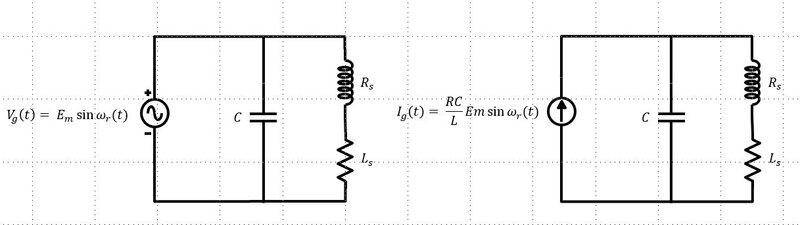I think even the circuit on the right should exhibit the same behavior as the first on the left (having voltage source): the impedance of the RLC network is the same ##Z(j\omega) = \frac{R_s + j\omega L_s}{(1+ j\omega CR_s - \omega^2L_sC)}## thus if we apply a current source ##I_g(t) = \frac {E_mR_sC} {L_s} sin (\omega_rt)## we get same results:

##i_c(t) = E_m\omega_rCcos(\omega_rt)##

##i_L(t) = \frac {E_mR_sC} {L}sin(\omega_rt) - E_m\omega_rCcos(\omega_rt)##

and thus the same expressions at AC steady-state for the instantaneous power delivered from the source and resistor dissipated power:

##P(t) = v_g(t)i_g(t) = \frac {E^2_mR_sC} {L_s}sin^2(\omega_rt)##

##W(t) = R_si^2_L(t) = \frac {E^2_mR_sC} {L_s}sin^2(\omega_rt - \phi)##

to me it seems the two cases are actually the same (even if in the applied current source case the transfer function involved has poles and zeroes exchanged w.r.t poles and zeroes of the applied voltage source case).

#### Attachments

Last edited:
I think even the circuit on the right should exhibit the same behavior as the first on the left (having voltage source): the impedance of the RLC network is the same ##Z(j\omega) = \frac{R_s + j\omega L_s}{(1+ j\omega CR_s - \omega^2L_sC)}## thus if we apply a current source ##I_g(t) = \frac {E_mR_sC} {L_s} sin (\omega_rt)## we get same results:
Help ! Even if in the network on the left (with voltage source) the two differential equations describing it are actually uncoupled, the behavior of the two networks is exactly the same, I think ! So, why call the network at right only "resonant system" while the other is not ?

ThanksLast edited:
So, why call the network at right only "resonant system" while the other is not ?
Because the Voltage Source across the C keeps Vc the same and C can store no 'extra' energy.

Because the Voltage Source across the C keeps Vc the same and C can store no 'extra' energy.
That's right, however in AC steady-state at resonant angular frequency ##\omega_r## the energy stored into C as function of time is the same as in the other case (current source case) as well as all others voltages and currents involved.

You forgot the Voltage Magnification in a resonant circuit. With a Voltage source you have a Zero source impedance across the C. No magnification.

You forgot the Voltage Magnification in a resonant circuit. With a Voltage source you have a Zero source impedance across the C. No magnification.
ok, got it. However voltage & current solutions of the system of (algebraic) differential equations (DAE) in the two cases (voltage source vs current source) are exactly the same (with no Voltage magnification in the voltage source case -- as you said). Consequently it seems to me that given an RLC network the concept of "resonance" actually relies on the applied source type (basically the transfer function involved is required to have at least a couple of complex coniugate poles).

Last edited:
the concept of "resonance" actually relies on the applied source type
Well - yes. The source Impedance is part of the circuit and the Q factor is forced to be unity. The source Impedance is frequently ignored in conversations about 'simple' resonances, mechanical as well as electrical.

Well - yes. The source Impedance is part of the circuit and the Q factor is forced to be unity. The source Impedance is frequently ignored in conversations about 'simple' resonances, mechanical as well as electrical.
That's my point of confusion...take the circuit from post #15 on the left (voltage source). We can calculate the circuit Q factor as:

##Q = 2\pi \frac {Max ~ energy ~ stored} {Power ~ loss}##

From previous posts the circuit Q at resonance angular frequency ##\omega_r = \sqrt { \frac 1 {L_sC} - \frac {R^2_s} {L^2_s} }## is:

##Q = \frac {\omega_rLs} {R_s}## or equivalently ##Q = \omega_rR_pC = \omega_r (\frac {L_s} {R_sC})C##

This value shouldn't be the unity, thought. 1 is just the value of voltage magnification (the fraction between Capacitor voltage and voltage source at resonance).

Last edited:
The source (a Voltage source) is equal to the voltage across any component in parallel with it. The Energy ‘in’ the C is v**2 C/2 because the PD has to be the same as the source PD.

The source (a Voltage source) is equal to the voltage across any component in parallel with it. The Energy ‘in’ the C is v**2 C/2 because the PD has to be the same as the source PD.
Sure, that basically means the voltage magnification is 1 however from that does not follow the circuit Q factor at resonance has to be 1.

Last edited:
So, for the latest incarnation of post #15: This is a version of the series LCR circuit, which you can see with the simple source transformation below. For LCR networks, you can always tell series from parallel by considering the resistance required for high Q. If it's small it's a series configuration, if it's large it's a parallel configuration.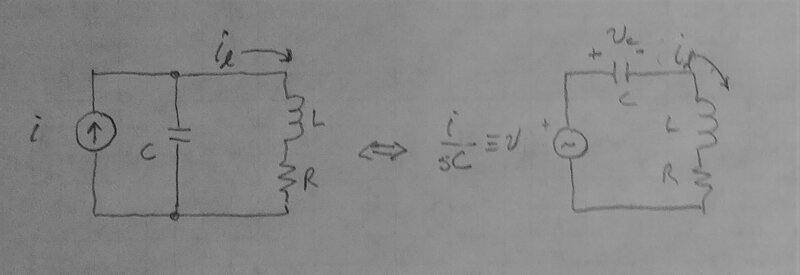You are correct that in steady state the total energy isn't constant, except when driven at resonance ## \omega = \frac{1}{\sqrt{LC}} ##.

Sometimes words aren't very efficient for this stuff. Have a look at the two solutions below. Both are steady state solutions where we assume that the circuit is lossy, so initial conditions don't matter, and the solution is a sinusoid at the driving frequency (linear networks don't make harmonics, etc.).

The first is the energy solution, which doesn't require solving for the current. The second is the solution for the current, which is a bit of a mess algebraically.

#### Attachments

• Series LCR energy.pdf
418 KB · Views: 99
• Series LCR soln.pdf
456.2 KB · Views: 102
Sure, that basically means the voltage magnification is 1 however from that does not follow the circuit Q factor at resonance has to be 1.
How are you defining Q?

View attachment 283292

You are correct that in steady state the total energy isn't constant, except when driven at resonance ## \omega = \frac{1}{\sqrt{LC}} ##.
Thanks for the reply and the job done.

I'm not sure to fully understand it (for instance the energy based solution). As far as I can understand what you've done is a Thevenin-based transformation of the pair current source - capacitor at a given angular frequency ##\omega## (we can actually choose ##\omega = \omega_0 = \frac{1}{\sqrt{LC}}
##).

That's good however I believe that transformation makes sense just in order to calculate currents/voltages of the resistor + inductor branch (Thevenin transformation actually applies to the "port" resistor + inductor branch is attached to). As a result the current through the capacitor used in your calculation (e.g. in the energy based solution) is not actually the same as the current through the capacitor in the circuit we started with. So I believe the value of the total stored energy as function of time in AC steady state even at angular frequency ##\omega_0 = \frac{1}{\sqrt{LC}}## does not stay actually constant in time.

What do you think about ?

Last edited:
How are you defining Q?
My definition of Q is the "classic" one - e.g. from wikipedia -- Q factor
It is basically the ratio between the (total) energy stored in the system and the energy dissipated in one cycle.

In the circuit under analysis (see post #15) total energy stored in the system (in the pair capacitor and inductor) at AC steady-state is not actually constant in time, though. Nevertheless we can pick the maximum value of the total stored energy and use it to calculate the Q factor as defined above.

My definition of Q is the "classic" one - e.g. from wikipedia -- Q factor
It is basically the ratio between the (total) energy stored in the system and the energy dissipated in one cycle
OK So the energy being put into the system, per cycle, is all dissipated in the system in one cycle. How is that not a Q of unity? I appreciate that the concept of a source impedance of zero is not real but it's only a limiting case.
Are you thinking that the impedance of the circuit that the Voltage source feeds can affect the source in some way? (i.e. one ideal thing beats another ideal thing?)

OK So the energy being put into the system, per cycle, is all dissipated in the system in one cycle. How is that not a Q of unity?
No, That's true just because a no "explosion" condition: the stored energy in the system (i.e. inside capacitor + inductor) cannot increment indefinitely in time thus energy being put from the source, per cycle, has to be equal to the energy dissipated per cycle.

I believe the stored energy involved in Q definition at numerator is actually the energy in the system at a given time t. It is not the energy being put in the system per cycle. As a result the Q value is not in general the unity (1).

Last edited:
This all seems lost in the semantic sea.May I ask the OP: what is the purpose of defining this particular parameter in this particular case? In my experience, the parallel RLC circuit (which is not exactly what you have sketched) is used mostly as a notch filter. As such the working definition of ##Q_{filter}## is typically related to the ratio of the bandwidth to the center freguency of the filter notch. Hence the "Q" of the filter. This definition is related to but not identical to the Q of a corresponding damped simple harmonic oscillator and instead indicates a ratio of dissipation to reaction in steady state.
The number of angels on the head of the pin is not the issue.

•sophiecentaur
Thanks for the reply and the job done.

I'm not sure to fully understand it (for instance the energy based solution). As far as I can understand what you've done is a Thevenin-based transformation of the pair current source - capacitor at a given angular frequency ##\omega## (we can actually choose ##\omega = \omega_0 = \frac{1}{\sqrt{LC}}
##).

That's good however I believe that transformation makes sense just in order to calculate currents/voltages of the resistor + inductor branch (Thevenin transformation actually applies to the "port" resistor + inductor branch is attached to). As a result the current through the capacitor used in your calculation (e.g. in the energy based solution) is not actually the same as the current through the capacitor in the circuit we started with. So I believe the value of the total stored energy as function of time in AC steady state even at angular frequency ##\omega_0 = \frac{1}{\sqrt{LC}}## does not stay actually constant in time.

What do you think about ?
Yes, you're correct, I missed that. The capacitor current is the difference between the source and the inductor current. There should be a frequency where the energy is constant however, since the ratio of capacitor and inductor current varies greatly with frequency change. As you said originally, the phase shift makes the calculation messy.

Yes, you're correct, I missed that. The capacitor current is the difference between the source and the inductor current. There should be a frequency where the energy is constant however, since the ratio of capacitor and inductor current varies greatly with frequency change. As you said originally, the phase shift makes the calculation messy.
I did the job to derive the expression for the total energy stored in post #15 network on the right (i.e. sinusoidal current source ##I_0##)

##E_L(t) = \frac {1} {2} L_sI^2_L(t)##, ##~E_C(t) = \frac {1} {2}CV^2_C(t)##, ##~I_L(t) = I_L sin (\omega t)##

##I_L = \frac {I_0} {(1 - ~\omega^2L_sC) ~+~ j\omega R_sC}##

##V_C(t) = I_L |R_s + j\omega L_s| sin (\omega t + \phi)##, ##~tan(\phi) = \frac {\omega L_s} {R_s}##

##E_L(t) = \frac {1} {2} \frac {I^2_0} {(1-~\omega^2 L_sC) ~+~ \omega^2 R^2_s C^2}L_ssin^2(\omega t)##

##E_C(t) = \frac {1} {2} \frac {I^2_0} {(1-~\omega^2 L_sC) ~+~ \omega^2 R^2_s C^2}C(R^2_s + \omega^2L^2_s)sin^2(\omega t + \phi)##

thus the total energy stored at time t is:

##E(t) = \frac {1} {2} \frac {I^2_0} {(1-~\omega^2 L_sC) ~+~ \omega^2 R^2_s C^2}[L_ssin^2(\omega t) + C(R^2_s + \omega^2L^2_s)sin^2(\omega t + \phi)]##

starting from this expression I do not think there exists actually an angular frequency ##\omega## the total stored energy stays constant in time.

Last edited:
The capacitor current is the difference between the source and the inductor current.
This is true, as K1 tells you but if the C is connected across a voltage source then the IC cannot be affected by the L. It's no longer a simple RLC resonant circuit so the concept of Q is not valid, I guess.
Q = CV so IC = C dV/dt
and
The Energy in the C is CV2/2 and is independent of dIL/dt
Unlike in a proper series RLC resonance, the Voltage across the RL is unaffected by the presence of the C.

I do not think there exists actually an angular frequency ω the total stored energy stays constant in time.
Yep. The existence of the Voltage source is upsetting the normal RLC situation because Energy can be supplied and removed by the source, independently of the Voltages across the L and C.

•cianfa72